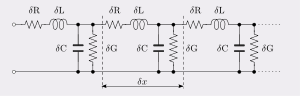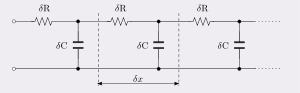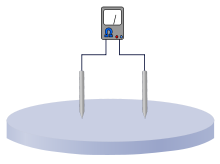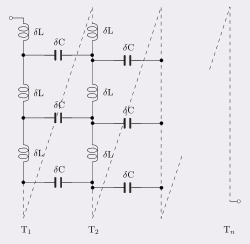# Distributed element model

Distributed element modelFig.1 Transmission line. The distributed element model applied to a transmission line.

In electrical engineering, the distributed element model or transmission line model of electrical circuits assumes that the attributes of the circuit (resistance, capacitance, and inductance) are distributed continuously throughout the material of the circuit. This is in contrast to the more common lumped element model, which assumes that these values are lumped into electrical components that are joined by perfectly conducting wires. In the distributed element model, each circuit element is infinitesimally small, and the wires connecting elements are not assumed to be perfect conductors; that is, they have impedance. Unlike the lumped element model, it assumes non-uniform current along each branch and non-uniform voltage along each node. The distributed model is used at high frequencies where the wavelength approaches the physical dimensions of the circuit, making the lumped model inaccurate.

## Applications

The distributed element model is more accurate but more complex than the lumped element model. The use of infinitesimals will often require the application of calculus whereas circuits analysed by the lumped element model can be solved with linear algebra. The distributed model is consequently only usually applied when accuracy calls for its use. Where this point is depends on the accuracy required in a specific application, but essentially, it needs to be used in circuits where the wavelengths of the signals have become comparable to the physical dimensions of the components. An often quoted engineering rule of thumb (not to be taken too literally because there are many exceptions) is that parts larger than one tenth of a wavelength will usually need to be analysed as distributed elements.

### Transmission lines

Transmission lines are a common example of the use of the distributed model. Its use is dictated because the length of the line will usually be many wavelengths of the circuit's operating frequency. Even for the low frequencies used on power transmission lines, one tenth of a wavelength is still only about 500 kilometres at 60 Hz. Transmission lines are usually represented in terms of the primary line constants as shown in figure 1. From this model the behaviour of the circuit is described by the secondary line constants which can be calculated from the primary ones.

The primary line constants are normally taken to be constant with position along the line leading to a particularly simple analysis and model. However, this is not always the case, variations in physical dimensions along the line will cause variations in the primary constants, that is, they have now to be described as functions of distance. Most often, such a situation represents an unwanted deviation from the ideal, such as a manufacturing error, however, there are a number of components where such longitudinal variations are deliberately introduced as part of the function of the component. A well known example of this is the horn antenna.

Where reflections are present on the line, quite short lengths of line can exhibit effects that are simply not predicted by the lumped element model. A quarter wavelength line, for instance, will transform the terminating impedance into its dual. This can be a wildly different impedance.

### High frequency transistorsFig.2. The base region of a bipolar junction transistor can be modelled as a simplified transmission line.

Another example of the use of distributed elements is in the modelling of the base region of a bipolar junction transistor at high frequencies. The analysis of charge carriers crossing the base region is not accurate when the base region is simply treated as a lumped element. A more successful model is a simplified transmission line model which includes distributed bulk resistance of the base material and distributed capacitance to the substrate. This model is represented in figure 2.

### Resistivity measurementsFig. 3. Simplified arrangement for measuring resistivity of a bulk material with surface probes.

In many situations it is desired to measure resistivity of a bulk material by applying an electrode array at the surface. Amongst the fields that use this technique are geophysics (because it avoids having to dig into the substrate) and the semiconductor industry also use it (for the similar reason that it is non-intrusive) for testing bulk silicon wafers. The basic arrangement is shown in figure 3, although normally more electrodes would be used. To form a relationship between the voltage and current measured on the one hand, and the resistivity of the material on the other, it is necessary to apply the distributed element model by considering the material to be an array of infinitesimal resistor elements. Unlike the transmission line example, the need to apply the distributed element model arises from the geometry of the setup, and not from any wave propagation considerations.

The model used here needs to be truly 3-dimensional (transmission line models are usually described by elements of a one-dimensional line). It is also possible that the resistances of the elements will be functions of the co-ordinates, indeed, in the geophysical application it may well be that regions of changed resistivity are the very things that it is desired to detect.

### Inductor windingsFig. 4. A possible distributed element model of an inductor. A more accurate model will also require series resistance elements with the inductance elements.

Another example where a simple one-dimensional model will not suffice is the windings of an inductor. Coils of wire have capacitance between adjacent turns (and also more remote turns as well, but the effect progressively diminishes). For a single layer solenoid, the distributed capacitance will mostly lie between adjacent turns as shown in figure 4 between turns T1 and T2, but for multiple layer windings and more accurate models distributed capacitance to other turns must also be considered. This model is fairly difficult to deal with in simple calculations and for the most part is avoided. The most common approach is to roll up all the distributed capacitance into one lumped element in parallel with the inductance and resistance of the coil. This lumped model works successfully at low frequencies but falls apart at high frequencies where the usual practice is to simply measure (or specify) an overall Q for the inductor without associating a specific equivalent circuit.

Wikimedia Foundation. 2010.

### См. также в других словарях:

• Distributed element filter — Figure 1. A circuit featuring many of the f …   Wikipedia

• Lumped element model — In general, the lumped component model is a way of simplifying the behaviour of spatially distributed systems into a topology consisting of discrete entities that approximate the behaviour of the distributed system under certain assumptions. It… …   Wikipedia

• Distributed GIS — concerns itself with GI Systems that do not have all of the system components in the same physical location. This could be the processing, the database, the rendering or the user interface. Examples of distributed systems are web based GIS,… …   Wikipedia

• Model-based design — (MBD) is a mathematical and visual method of addressing problems associated with designing complex control, signal processing and communication systems. It is used in many motion control, industrial equipment, aerospace, and automotive… …   Wikipedia

• Distributed operating system — A distributed operating system is the logical aggregation of operating system software over a collection of independent, networked, communicating, and spatially disseminated computational nodes. Individual system nodes each hold a discrete… …   Wikipedia

• Model–view–controller — A general representation of the MVC design pattern. Model view controller concept. The solid line represents a direct as …   Wikipedia

• Model based design — The dawn of the electrical age brought with it various novel, innovative and advanced control systems. It was as early as 1920 s when the two strands of technology, control theory and control system, came together to produce large scale… …   Wikipedia

• Electrical element — Electrical elements are conceptual abstractions representing idealized electrical components, such as resistors, capacitors, and inductors, used in the analysis of electrical networks. Any electrical network can be analysed as multiple,… …   Wikipedia

• Network element — A network element is usually defined as a manageable logical entity uniting one or more physical devices. This allows distributed devices to be managed in a unified way using one management system. According to Telecommunications Act of 1996, the …   Wikipedia

• Common Diagnostic Model — The Common Diagnostics Model, or CDM, is a diagnostics standard developed and maintained by the Distributed Management Task Force (DMTF). CDM models the entire flow of diagnosis from test discovery, configuration and execution to progress updates …   Wikipedia

### Поделиться ссылкой на выделенное

##### Прямая ссылка:
Нажмите правой клавишей мыши и выберите «Копировать ссылку»